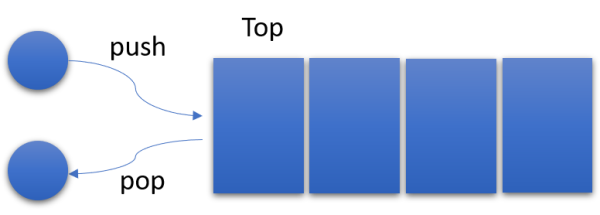## Data structures, the humble Stack

The stack is pretty much one of those simple data type abstraction types that most of us give for granted, I mean, most developers that I know will probably answer back many situation or cases where they need to use a queue but not when and why did they use a stack. It is a very simple and humble structure, much more simplest than a queue (but very similar) and as with its friend the queue, it can be implemented in many different ways using separate data structures (a stack is an abstract data type).

The idea is pretty simple, a stack is a LIFO structure and it looks more like those pile of papers you have on your desk waiting to be classified, the one at the top are the first you are going to look at. Under that concept we can say we can add or remove elements only from and to the head of the stack, so we only need one variable to point which is that element in the stack.This sounds like a really good place to use a linked list, not a full linked list if you think about it, because we only need to prepend data to list or remove data from the top, so we can use the same principle to implement a simple and humble stack (this time in Kotlin), notice thanks to its simplicity and way of work every operation in our stack will be always $$\Theta(1)$$:

import java.util.*

class Stack<T> {
private var top: Node? = null
private inner class Node(val value: T, val next: Node?)

fun push(element: T) {
top = Node(element, top)
}

fun pop(): T {
val result = top?.value ?: throw EmptyStackException()
top = top?.next
return result
}

val isEmpty: Boolean
get() = top == null
}


You could implement a fixed size stack easily using an array (very similar to what we did with a queue) and you will see it won’t need anything complex (like a chasing pointer) as we had to do with the queue. I left you to the homework to do a simple limited size stack, remember to have fun!

As usual I left you with the unit test for this queue, this time using kotlin.test:

import java.util.*
import kotlin.test.*

class StackTests {
@Test
fun Just created stack is empty() {
val stack = Stack<Int>()
assertTrue { stack.isEmpty }
}

@Test
fun Stack can push and pop() {
val stack = Stack<Int>()
stack.push(1)
stack.push(2)

assertFalse { stack.isEmpty }
assertEquals(2, stack.pop())
assertFalse { stack.isEmpty}
assertEquals(1, stack.pop())
assertTrue { stack.isEmpty }
assertFailsWith(EmptyStackException::class) {
stack.pop()
}
}
}